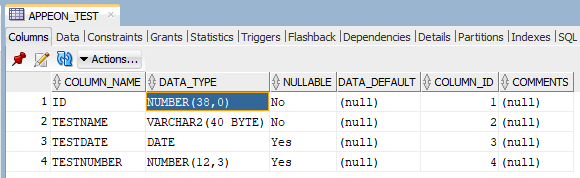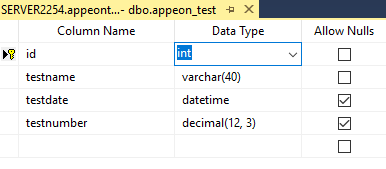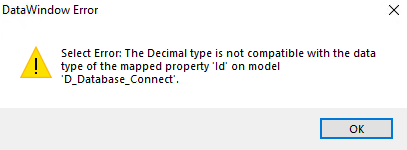### Different execution results in different databases

Supposing we are executing the following CREATE TABLE syntax in SQL Server and Oracle.

`CREATE TABLE appeon_test (id integer NOT NULL,testname varchar(40) NOT NULL , testdate date  , testnumber decimal(12,3)  , PRIMARY KEY (id)) `

With Oracle, the table created is as below:With SQL Server, the table created is as below:In the PowerBuilder DataWindow SRD, the datatype is long, which can work well in both databases.

`table(column=(type=long update=yes updatewhereclause=yes key=yes name=id dbname="appeon_test.id" )`

When converting the DataWindow to C# model, with SQL Server, the Id column is of int type:

```[DwColumn("appeon_test", "id")]
public int Id { get; set; }
```

Because the Id column of the table is Number in Oracle, when the same model tries to retrieve data from the Oracle database, an error occurs:Therefore, if using the Oracle database, the model Id shall be changed to the decimal data type.

```[DwColumn("appeon_test", "id")]
public decimal Id { get; set; }
```

If you hope to run the same model against different databases, it is necessary to add ValueConverter too the model column in the .cs file by:

```[ValueConverter(typeof(DefaultValueConverter))]
[DwColumn("appeon_test", "id")]
public int Id { get; set; }
```